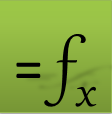# Solving Nonlinar Equations Videos

## Description

This video demonstrates how to use the function NLSOLVE to solve nonlinear equation systems in both Excel and Google Sheets. Multiple examples are illustrated including a system with inequality equation and a parameterized integral problem. NLSOLVE is based on Levenberg, Marquardt algorithm.

## Description

This video demonstrates how to use the function NLSOLVE to find least-squares best fitting curve for a set of (x,y) data points in both Excel and Google Sheets. NLSOLVE is based on Levenberg, Marquardt algorithm.
Question or Comment? Email us:
support @ excel-works.comExceLab: Transforming Excel into a Calculus Power House

ExceLab functions and methods are protected by USA Patents 10628634, 10114812, 9892108 and 9286286.